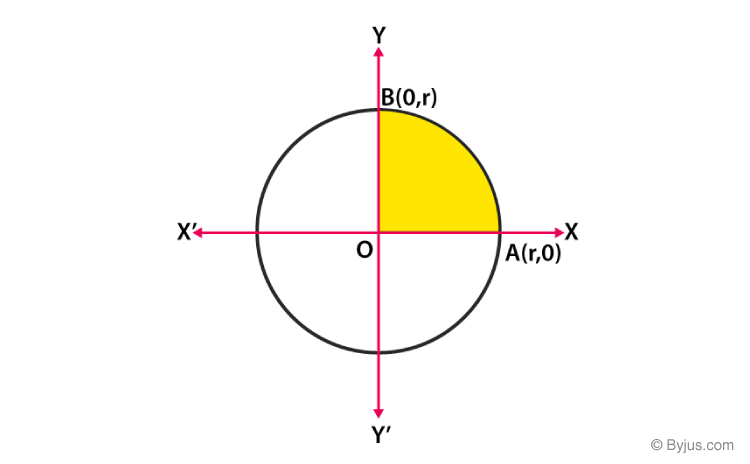# Application of Integrals

There is a number of methods of calculations among which are functions, differentiation and integration. Application of Integrals is applied in various fields like Mathematics, Science, Engineering etc. For the calculation of areas, we use majorly integrals formulas. So let us give here a brief introduction on integrals based on the Mathematics subject to find areas under simple curves, areas bounded by a curve and a line and area between two curves, and also the application of integrals in the mathematical disciplines along with the solved problem.

## Integral Definition

An integral is a function, of which a given function is the derivative. Integration is basically used to find the areas of the two-dimensional region and computing volumes of three-dimensional objects. Therefore, finding the integral of a function with respect to x means finding the area to the X-axis from the curve. The integral is also called as anti-derivative as it is the reverse process of differentiation.

## Types of Integrals

There are basically two types of integrals, Definite and Indefinite. Definite Integral is defined as the integral which contains definite limits,i.e., upper limit and lower limit. It is also named as Riemann Integral. It is represented as;

$$\begin{array}{l}\int_{a}^{b}f(x)d(x)\end{array}$$

Indefinite Integral is defined as the integral whose upper and lower limits are not defined.

$$\begin{array}{l}\int f(x)d(x)=F(x)+C \text{ is defined as the indefinite integral, where C is the constant value.}\end{array}$$

## Application of Integrals

There are many applications of integrals, out of which some are mentioned below:

In Maths

• To find the centre of mass(Centroid) of an area having curved sides
• To find the area between two curves
• To find the area under a curve
• The average value of a curve

In Physics

Integrals are used to calculate

• Centre of gravity
• Mass and momentum of inertia of vehicles
• Mass and momentum of satellites
• Mass and momentum of a tower
• The centre of mass
• The velocity of a satellite at the time of placing it in orbit
• The trajectory of a satellite at the time of placing it in orbit
• To calculate Thrust

## Video Lesson

### Area under the curve### Important Questions### Definite Integral Problem

Let us discuss here how the application of integrals can be used to solve certain problems based on scenarios to find the areas of the two-dimensional figure.

Example: Find the area enclosed by the circle x2+y2=r2, where r is the radius of the circle.

Solution: Let us draw a circle in the XY plane with a radius as r.From the graph,

OA=OB=r

A has coordinates(0,r) on the x-axis and B has coordinates(r,0) on y-axis.

Area of Circle=4*Area of region OBAO

$$\begin{array}{l}\text{Area of Circle= } 4*\int_{0}^{r}y.dx\end{array}$$

Now, from the equation of circle,

x2+y2=r2

y2=r2-x2

y=r2-x2

The region OABO lies in the first quadrant of the x-y plane.

$$\begin{array}{l}\text{Therefore, y= }\pm \sqrt{r^2-x^2}\end{array}$$
$$\begin{array}{l}\text{Now we can write, Area of circle = }4*\int_{0}^{r}\sqrt{r^2-x^2}.dx\end{array}$$

From the differentiation formula,

$$\begin{array}{l}\sqrt{r^2-x^2}.dx=\frac{1}{2} \sqrt{r^2-x^2}+ \frac{r^{2}}{2}sin^{-1}\frac{x}{4}+c\end{array}$$

Therefore,

$$\begin{array}{l}=4\left [ \frac{x}{2}\sqrt{r^{2}-x^{2}} + \frac{r^{2}}{2} sin^{-1}\frac{x}{r}\right ]^{r}_{0}\end{array}$$
$$\begin{array}{l}=4\left [ \frac{r}{2}\sqrt{r^{2}-r^{2}} + \frac{r^{2}}{2} sin^{-1}\frac{r}{r} – \frac{0}{2}\sqrt{r^{2}-0}-\frac{0^{2}}{2}sin^{-1} (0)\right ]\end{array}$$
$$\begin{array}{l}=4\left [ 0 + \frac{r^{2}}{2}sin^{-1}(1)-0-0\right ]\end{array}$$
$$\begin{array}{l}=4.\frac{r^{2}}{2}.\frac{\pi }{2}\end{array}$$
$$\begin{array}{l}\text{Area of circle}=\pi r^{2}\end{array}$$